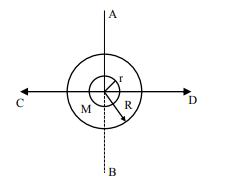# The figure shows two solid discs with radius R and r respectively.Question:

The figure shows two solid discs with radius R and r respectively. If mass per unit area is same for both, what is the ratio of MI of bigger disc around axis AB (Which is  to the plane of the disc and passing through its centre) of MI of smaller disc around one of its diameters lying on its plane? Given 'M' is the mass of the larger disc. (MI stands for moment of inertia)1. $\mathrm{R}^{2}: \mathrm{r}^{2}$

2. $2 r^{4}: R^{4}$

3. $2 R^{2}: r^{2}$

4. $2 R^{4}: r^{4}$

Correct Option: , 4

Solution:

Ratio of moment of inertia $=\frac{\frac{1}{2} \mathrm{MR}^{2}}{\frac{1}{4} \mathrm{mr}^{2}}$

$=\frac{2 \sigma \pi \mathrm{R}^{2} \mathrm{R}^{2}}{\sigma \pi \mathrm{r}^{2} \mathrm{r}^{2}}=\frac{2 \mathrm{R}^{4}}{\mathrm{r}^{4}}$